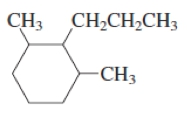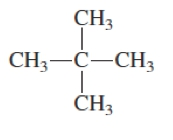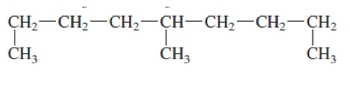## General Chemistry

Learn the toughest concepts covered in Chemistry with step-by-step video tutorials and practice problems by world-class tutors

22. Organic Chemistry

# Naming Alkanes

As with any functional group there is a list of rules that must be followed for naming alkanes.

Naming Alkanes
1
concept

## Rules for Naming Alkanes3m
Play a video:
Hey, guys. So here we're gonna go over some basic rules. Help us name out cane structures. So we say when naming any out king compound. These are the sets of rules that we're going to follow. Rule number one. We find the longest carbon chain and assign a route name accordingly. So if you're changing six carbons long, it's heck saying, If it's eight carbons long, it's octane. If there is a tie between longest chains, choose the chain that gives the most substitute substitue INTs. So if you have to change both or five, but one has three substitutes, and the other one only has one go with the one that gives you three substitutes. Number the chain from the end closest toe. One of these substitutes Next substitutes will be named alphabetically, and a number will accompany the location of their attachment. So if we have a method on carbon to, it'll lead to metal. If more than one of a similar out he'll group, which is a substitute, went is present. Now we must use numerical prefixes to describe the number of them. So if we have two methods of the dime ethel or it could be Try metal tetra method if there's four of them and then Penta metal. So here we look at two structures. They're the same structure we have to identify. Which one is the correct, uh, name from the structure giving so the longest chain. And this one looks like it could be here. And when we looked at 10. And then here. This will also be 10. So both have 10 carbon. So both have the same length for the longest carbon chain. We go with the one that gives us the most substitutes. Substitutes are the ones that are not part of the longest chain. So this is one substitute, Wint. And then this whole thing would be to this one. Here is one substitute went two substitutes 34 So the second structure would be the better structure because it gives us more substitutes. So now we numbered from the end closest to one of these substitutes. He would have to go 12345 before we get to a substitue int. Or we could just go 12 and we're already at our substitute. So and 10 Again. The route name would be decade because it's 10 carbons. Here we have two carbons in this substitution. So this is Ethel. This one here has four carbons in the substitution. So this is beautiful. This one has one carbon. So it's metal, and this one also is method. So what would the name be? What we said The route name is decade. We named the substitutes alphabetically. So be before e before em so bugles on carbon five. So this would be five beautiful apples on carbon 66 Ethel and then on carbons two and four, we have metals. So we say to come before di method and again And the name is decade. So dimethyl decade is all one word. No space in between them. So this would be the official name of the structure. This way of numbering is correct. This one is not because it doesn't give us as many substitute groups2
example

## Naming the following alkane compound2m
Play a video:
Hey, guys, In this new video, we're gonna continue with our discussion of naming Al Canes. So in this first one, we're asked to name the following Alcan compound. What we need to realize here is that here we have a rank. So this is a cyclic al cane and because it's in a ring, we say cyclo. And here it has six carbon. Because remember, every edge, every corner is a carbon. So there's six carbons within this rink, so it's gonna be called cyclo because it's cyclic. Six carbons is heck sane. So this is a cyclo hexane. Now you wanna number this? Um, you wanna number the carbons in the ring in a way that these groups, which are substitue INTs which didn't get numbered, get the lowest numbers possible? What does that mean? That means that I want to count either. 4123 He really doesn't matter because it's symmetrical both ways. So let's go with the first way we're gonna stay here, that this is one carbon a ch three. So this is a metal. So again it becomes a point that you guys remember what these groups look like here. This is three carbons connected to something. So this is a probable And again this is a method, so m before p so alphabetical order for substitue INTs. There are two metals, so that's dimethyl. They're located on carbons one in three. So this will be 13 dime Ethel and notice how the numbers are grouped together and separated by a comma. But numbers and words are separated by a hyphen. And then the propose on carbon to so hyphen to hyphen because that propose on carbon to two pro pool cyclo heck, sane. And that should all be one word. So I'm gonna move it closer. So that would be the name off our compound and again because we're dealing with a ring, we use the word cyclone now. So now that we've attempted this one, take a look at the next one. And once you figure out what it could possibly be named, click on the next video and see an example of me going over it and see if your answer matches mine3
example

## Naming the following alkane compound1m
Play a video:
Alright, guys, let's continue with the naming of Al Keynes. So here in this next example, it's got a name, this structure. So what we gotta do here is look for the longest chain so you can go. 123 you go. 123 You go. 123 It doesn't matter which way you count. The longest chain is three carbons. So let's just make it simple. Ourselves will say that this is the longest chain, which means these two things here are substitue INTs. So that's a method here and a method here. And we want to count from the end one of the end. So we could say 123 or 123 the other way, we count from the end closest to the substitue INTs. Either way gets me there on carbon too. Now here's the thing. There's two methods, so that's dimethyl. Now I know they're both on the same carbon, but you can't say to dimethyl because to dimethyl means this to die. Method just means okay, there are two method groups and I know the location of one of them. One of them's on carbon too. But I don't know what the location of the other one is. So you have to actually say to to dimethyl, you have to state to twice, Okay, because each one is its own separate thing, located on carbon, too. We use die to pull them together because they have similar names. But each one is. It's an individual substitution. So we have to to dimethyl. And a three carbon chain is propane, and that's what we have named the structure. So keep practicing. Keep going all over all the different examples that we're going through. So attempted Next one click on the next video and see how I answer it and check to see if your answer matches mine.4
example

## Naming the following alkane compound1m
Play a video:
Alright, guys, let's take a look at the example that was left for us in terms of naming. So this was a long structure, remember? Look for the longest carbon chain, the longest carbon chain is obviously this chain here. Remember, you want to count from the end closest to the substitue int whatever didn't get counted, which in this case, is that metal counting from either way gets us there. Because if you go here 12345 carbon five would have substitue int if you go here. 12345 again it has a substitution. So numbering either way is fine. So we'll say this is 678 and nine nine Carbons is called no name and the metal group is on carbon number five. So this one was not as bad as the previous two. So this would just be five metal known. So we've gone over different scenarios. Go to the next page and see if you can tackle the next naming set. Use the same principles that we have applied now to name the next group of compounds, come back and see how I approach them
5
example

## Determine the structure for the following alkane compound.3, 4, 5-trimethyldecane1m
Play a video:
alright, guys in these next examples were given the name of the compound and asked to dry here. This may seem like a harder process, but actually drawing the compound from the name is actually easier. What you should do is start with the end of the name. The end of the name forms the chain, the base and we're gonna say here we have decade, which is 10 carbons. So ch three siege to siege to. So the hard part is writing all this out. Ch two ch two ch two c h eight, nine and then 10. Now on carbons 34 and five. There are metals, So 10. Now what you should notice you're at the moment is every single carbon is making four bonds. So when I put on those metal groups on carbons 34 and five, those carbons would be making five bonds too many bonds. So how do they compensate for this extra metal group? They lose Ah, hydrogen. So in carbon three with a race and H so that we can accommodate that incoming method group. Same thing for carbon four and same thing with carbon five. Okay, so that would be our structure. And there's a bomb between them. Okay, so we lose an HF of each of them so that we can accommodate that incoming method group. So this is something you need to remember. Carbon is Tetra Valent can't go beyond four bonds. That was key to getting the correct answer here. Now that we've done that, go to the next one again. Start with the end of the name that forms the chain itself and then place the substitution on the right carbon and you're done.
6
example

## Determine the structure for the following alkane compound.4-tert-butyloctane1m
Play a video:
Alright guys, let's continue with naming about Keynes. So in this example here we have to determine the structure for the following out King compound. So it's four ter beautiful octane. So octane means a carbons. So in a carbon chain, keep writing So 12345678 Carbons number at 1234567 and eight. Carbon number four is going to have a terp, Udal. So it has to lose an H to accommodate that incoming ter bugle. Remember, ter beautiful would look like this. It's a carbon connected and it has three methyl groups on it. So that would be our four ter beautiful octane. They find the last question guys attempt to do this one. This one has PAL Agence as thes substitue INTs. So flooring is floral. Chlorine is chloral Brahman is bro mo eyes I Odo I Odiah. So knowing this is key to answering the next question
7
example

## Determine the structure for the following alkane compound1m
Play a video: